# Subtract a Value from every Number in a List in PythonLast updated: Feb 23, 2023
4 min## #Subtract a value from every number in a List in Python

To subtract a value from every number in a list:

1. Use a list comprehension to iterate over the list.
2. Use the subtraction `-` operator to subtract a value from every number.
3. The new list will contain the results.
main.py
```Copied!```a_list = [5, 15, 25, 35, 45]

a_list = [item - 5 for item in a_list]

print(a_list)  # 👉️ [0, 10, 20, 30, 40]
``````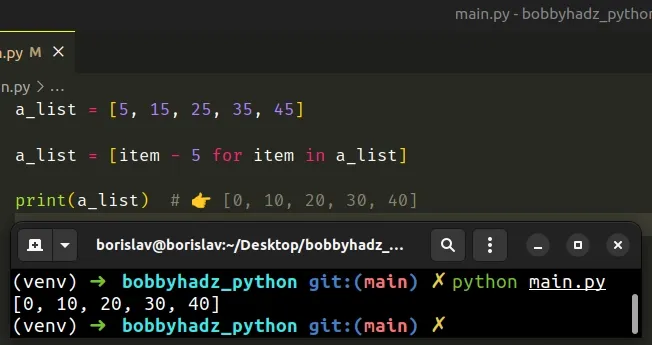We used a list comprehension to iterate over the list and subtracted `5` from each number.

List comprehensions are used to perform some operation for every element, or select a subset of elements that meet a condition.

On each iteration, we subtract `5` from the current list item and return the result.

Alternatively, you can use a simple `for` loop.

## #Subtract a value from every number in a List using a `for` loop

This is a four-step process:

1. Declare a new variable that stores an empty list.
2. Use a `for` loop to iterate over the original list.
3. On each iteration, subtract the number from the current item.
4. Append the result to the new list.
main.py
```Copied!```a_list = [5, 15, 25, 35, 45]

new_list = []

for item in a_list:
new_list.append(item - 5)

print(new_list)  # 👉️ [0, 10, 20, 30, 40]
``````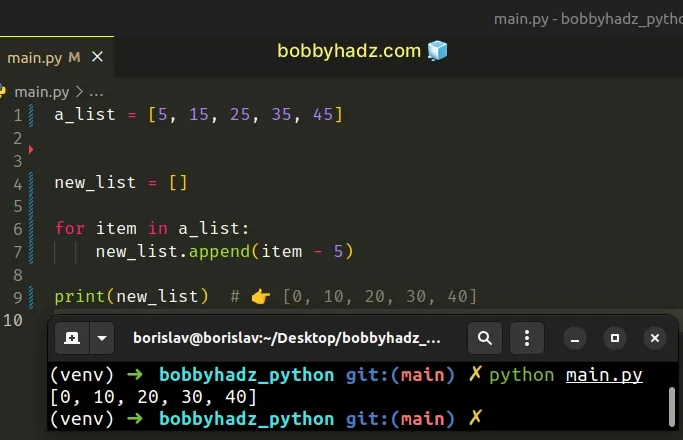The `for` loop works in a very similar way to the list comprehension, but instead of returning the list items directly, we append them to a new list.

The list.append() method adds an item to the end of the list.

## #Subtract a value from every number in a List using NumPy

If you use the `numpy` module, you can also subtract the number directly from a `numpy` array.

main.py
```Copied!```import numpy as np

arr = np.array([5, 15, 25, 35, 45])

arr = arr - 5

print(arr)  # 👉️ [ 0 10 20 30 40]

a_list = arr.tolist()
print(a_list)  # 👉️ [0, 10, 20, 30, 40]
``````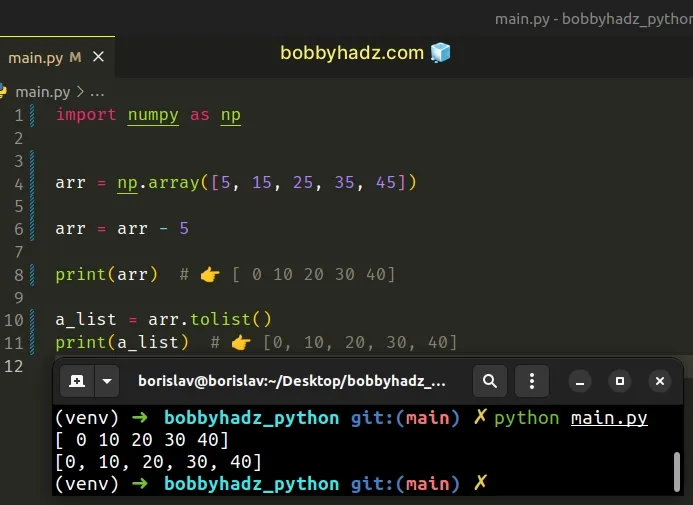Make sure you have NumPy installed to be able to run the code sample.

shell
```Copied!```pip install numpy

# 👇️ or with pip3
pip3 install numpy
``````

Subtracting a number from a `numpy` array effectively subtracts the number from each element in the array.

Note that this only works with `numpy` arrays, you'd get a `TypeError` if you try to subtract a number from a native Python list.

The tolist method converts a `numpy` array to a list.

Alternatively, you can use the `map()` function.

## #Subtract a value from every number in a List using `map()`

This is a three-step process:

1. Pass a lambda function and the list to the `map()` function.
2. The lambda function should subtract the specific value from the provided list element.
3. Use the `list()` class to convert the map object to a list.
main.py
```Copied!```a_list = [5, 15, 25, 35, 45]

new_list = list(map(lambda item: item - 5, a_list))

print(new_list)  # 👉️ [0, 10, 20, 30, 40]
``````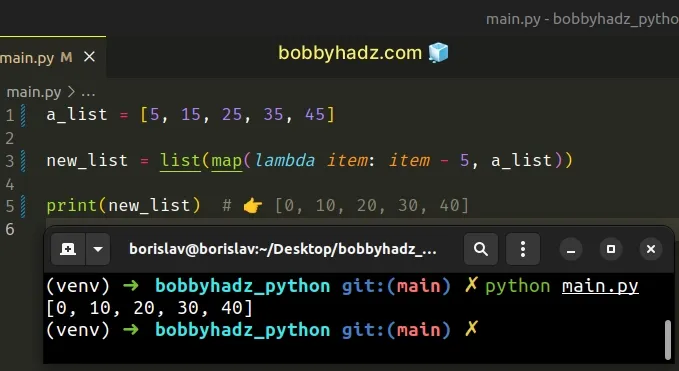The map() function takes a function and an iterable as arguments and calls the function with each item of the iterable.

The lambda function we passed to `map` gets called with each item in the list, subtracts `5` from the item and returns the result.

The last step is to use the `list()` class to convert the `map` object to a `list`.

Which approach you pick is a matter of personal preference. I'd use a list comprehension because I find them quite direct and easy to read.

## #Subtract a value from every number in a List using `enumerate`

You can also use the `enumerate()` function to subtract a value from every number in a list.

main.py
```Copied!```a_list = [5, 15, 25, 35, 45]

for index, item in enumerate(a_list):
a_list[index] = item - 5

# 👇️ [0, 10, 20, 30, 40]
print(a_list)
``````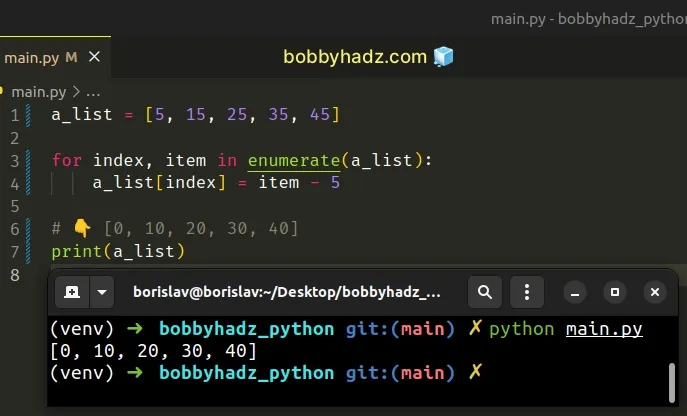The enumerate function takes an iterable and returns an enumerate object containing tuples where the first element is the index and the second is the corresponding item.

main.py
```Copied!```my_list = ['bobby', 'hadz', 'com']

for index, item in enumerate(my_list):
print(index, item)  # 👉️ 0 bobby, 1 hadz, 2 com
``````

On each iteration, we use the `index` to assign to the current list item, subtracting `5` from its value.

I've also written an article on how to sum all values in a dictionary or list of dictionaries.